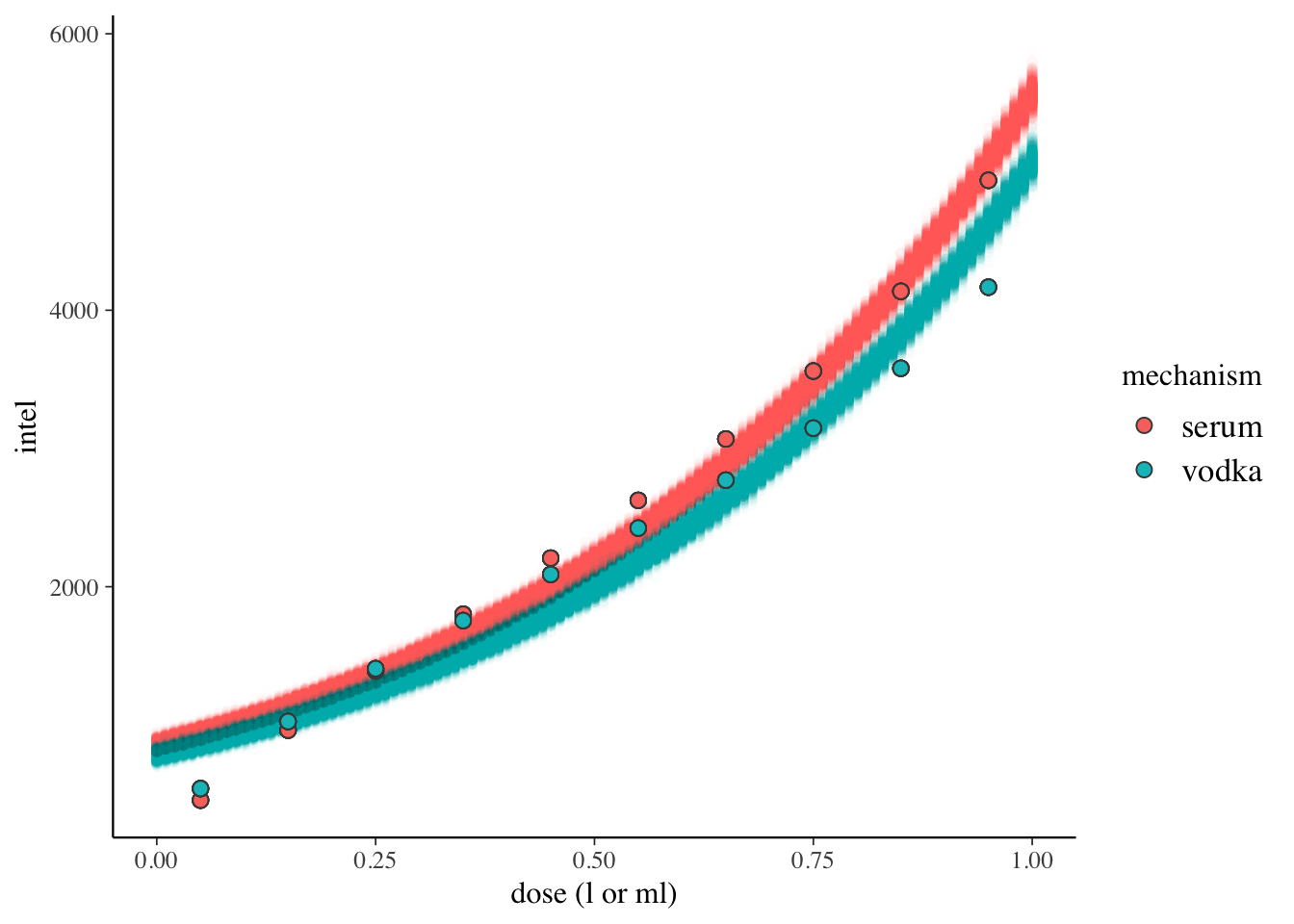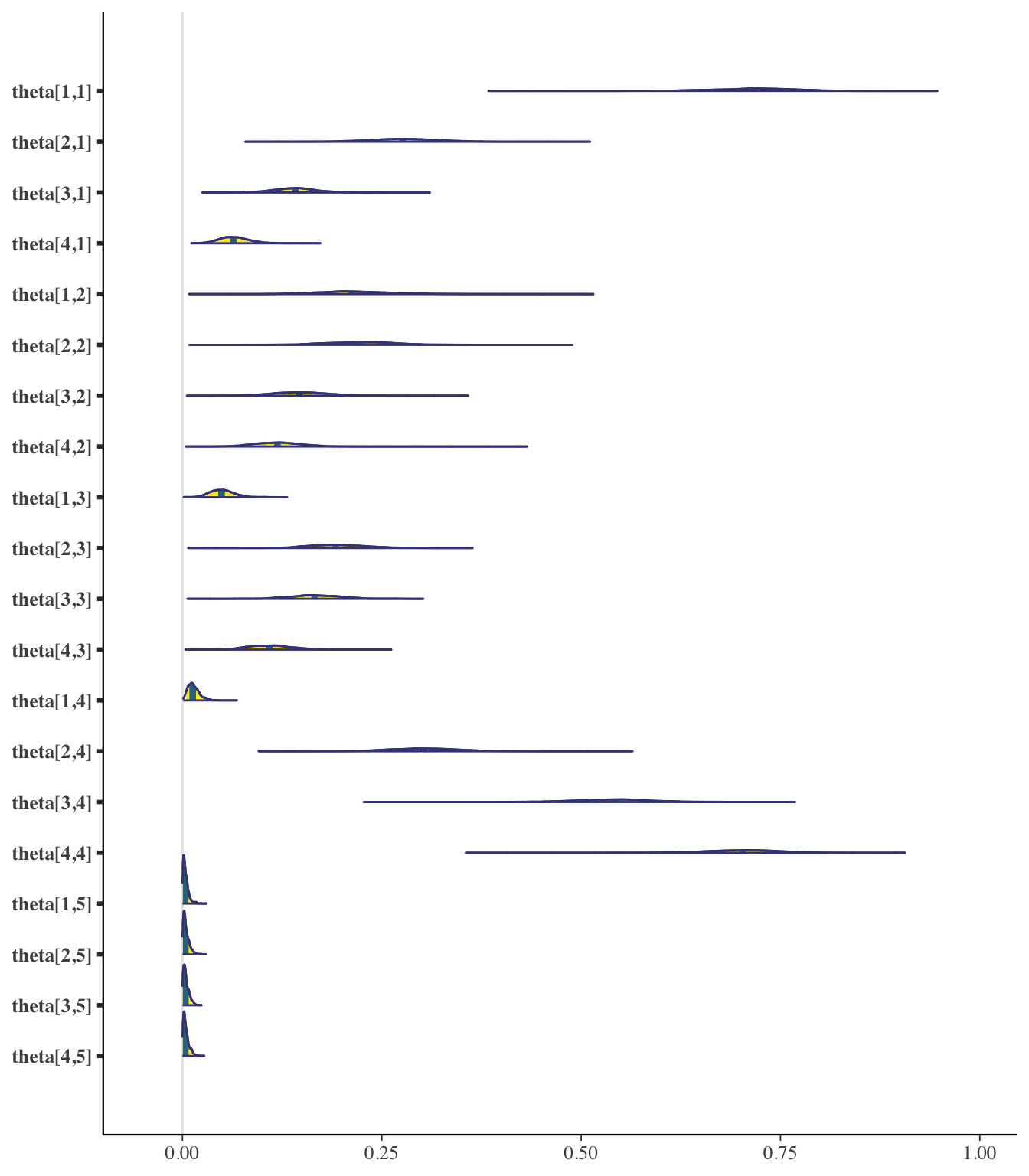This notebook is just for fun and a way of testing out some of the power of Stan.

Let’s say we work for the CIA and want to determine if a truth serum or vodka works better for getting tips and valuable information from criminal Russian agents we interrogate. How would we test the effectiveness of the two treatments? Let’s try a few models and see if we can figure out which one will get us the intel we need..

``````  set.seed(123)
needs('rstan')
needs('rstanarm')
needs('tidyverse')
needs('BayesDA')
needs('bayesplot')
needs('brms')
source('helpers.R')

# model output exceeds default print
options(max.print=1E4)

# prevent tedious re-compilation during interactive Stan dev
rstan_options(auto_write = TRUE)

# use multiple cores during sampling
options(mc.cores = parallel::detectCores())``````

We simulate some data of the effectiveness of the vodka and serum. We quantify how many useful tips and pieces of intel we gather per ml of dose.

``````  # prior effectiveness CIA operation with vodka/serum

#litres
vodka <- rep(seq(0.05, 0.98, by=0.1), 8)
#ml
serum <- rep(seq(0.05, 0.98, by=0.1), 8)
# quantity of intel
intelVodka <- floor(sapply(vodka, function(q) { (qbeta(q, 6, 2) * 17)^3 }))
intelSerum <- floor(sapply(serum, function(q) { (qbeta(q, 6, 2) * 12)^3.5 }))
intelResults <- bind_rows(                                 # dplyr style stacks
tibble(mechanism="serum",  dose=serum,  intel=intelSerum),
tibble(mechanism="vodka", dose=vodka, intel=intelVodka)) %>% mutate(treatment = ifelse(mechanism == 'serum', 1, 0))``````

We start simple with a poisson glm of the counts of useful pieces of intel for every serum drop. This assumes a constant rate of intel for every bottle of vodka, which actually might make sense for our Russian agents who seem impervious to the law of diminishing marginal utility.

``````  # we can model the prior and the likelihood as  ax + b logit
# then use the poisson
# p.75
# p.124.. meta analysis of studies
fitIntel <- stan_glm(intel ~ dose + factor(treatment), family=poisson, data=intelResults)``````
``````  newdata <- bind_rows(                                 # dplyr style stacks
tibble(mechanism="serum", dose=seq(0, 1, by=0.01)),
tibble(mechanism="vodka", dose=seq(0, 1, by=0.01))) %>% mutate(treatment = ifelse(mechanism == 'serum', 1, 0))

stan.mu <- posterior_predict(fitIntel, newdata, draws = 1000)
newdata <- data.frame(intel = c(stan.mu),
mechanism = rep(newdata\$mechanism, each = 1000), treatment = rep(newdata\$mechanism, each = 1000),
dose = rep(newdata\$dose, each = 1000), treatment = rep(newdata\$treatment, each = 1000))

print( fitIntel)``````
``````## stan_glm
##  family:       poisson [log]
##  formula:      intel ~ dose + factor(treatment)
##  observations: 160
##  predictors:   3
## ------
## (Intercept)        6.7    0.0
## dose               1.9    0.0
## factor(treatment)1 0.1    0.0
##
## Sample avg. posterior predictive distribution of y:
## mean_PPD 2402.7    5.5
##
## ------
## * For help interpreting the printed output see ?print.stanreg
## * For info on the priors used see ?prior_summary.stanreg``````

Our fit, even still, is not great. We’re way off in the lower/upper quartiles and our predictions aren’t very useful.

``````  alpha_level <- .01

ggplot() +
geom_point(alpha=alpha_level, data=newdata, aes(color=mechanism, x=dose,y=jitter(intel))) +
geom_point(alpha=1, stroke=0.5, data=intelResults, aes(x = dose, y = intel, fill = mechanism), colour="#474747", pch=21, size=2.5) +
labs(x = 'dose (l or ml)', y = 'intel')``````So we decide to contact an embedded agent we know who has run a survey on Russians of which vodka they prefer. We have data broken down by age, region and vodka brand. We can use this to see if we can figure out why our vodka data seems so noisy. Maybe soe Russians like one vodka more than another?

``````  # we decide to run a survey to determine which vodka to use, to improve our model further
# multinomial model p. 70
# 209
vodkas <- c('Stoli', 'Absolut', 'Smirnoff', 'Green Mark')
nVodkas <- length(vodkas)

# Z districts
rDistricts <- c('Central', 'Northwest', 'Siberia', 'Ural')

# J types of Russians
yService <- c('new', 'jr', 'mid', 'vet')
# factor dummy vars
servicei <- c(1L,2L,3L,4L)
# K clusters for vodkas, with J types in each
vodkaCountStoli <- c(50L,40L,50L,60L,  30L, 15L, 30L, 10L,  20L, 5L, 13L, 8L,  8L, 3L, 2L, 6L)
vodkaCountAbsolut <- c(20L, 20L, 20L, 3L,  20L, 40L, 11L, 5L,  20L, 10L, 10L, 8L,  8L, 8L, 5L, 14L)
vodkaCountSmirnoff <- c(4L, 4L, 4L, 2L,  20L, 12L, 20L, 7L,  20L, 13L, 6L, 14L,  3L, 5L, 4L, 20L)
vodkaCountGreenMark <- c(1L, 1L, 1L, 1L,  25L, 25L, 30L, 15L,  65L, 30L, 40L, 30L,  45L, 55L, 40L, 50L)

alphaVodka <- rep(1/nVodkas, nVodkas)
Stoli=vodkaCountStoli,
Absolut=vodkaCountAbsolut,
Smirnoff=vodkaCountSmirnoff,
GreenMark=vodkaCountGreenMark,
None=rep(c(0L),16),
district=rep(servicei,4))

vodkaI <- c(rep(c(rep(c(1L),4), rep(c(2L),4), rep(c(3L),4), rep(c(4L),4)),5))

vodkaIndex= vodkaI,
vodkaPick=c(rep(c(0L),16), vodkaCountStoli, vodkaCountAbsolut, vodkaCountSmirnoff, vodkaCountGreenMark),
district=rep(servicei,20))

J_age <- n_levels(idx_age)
J_district <- n_levels(idx_district)

y <- vodkaData %>% select(Stoli, Absolut, Smirnoff, GreenMark, None) %>% data.matrix()
K <- ncol(y)

G <- 2

maxResponse <- 100
z <- 400``````

We can construct a hierarchical multinomial model in Stan to learn more about which age groups prefer which vodka. That way, we can give our agents the vodkas they prefer.

It turns out younger seemingly-Hipster agents are throwing our data off! They prefer Stoli while older agents prefer a cheap vodka. Our confidence intervals are wide but we can use this data.

``````   vh_path <- "./3/multinomialhierarchy_ari.stan"
v2 <- stan_model(vh_path)

fitVodkaH <- sampling(v2, chains=4, iter = 500, verbose = TRUE, save_warmup = FALSE, control = list(adapt_delta = 0.95)) ``````
``````##
## CHECKING DATA AND PREPROCESSING FOR MODEL 'multinomialhierarchy_ari' NOW.
##
## COMPILING MODEL 'multinomialhierarchy_ari' NOW.
##
## STARTING SAMPLER FOR MODEL 'multinomialhierarchy_ari' NOW.``````
``````  #as.matrix(fitVodkaH)
bayesplot::color_scheme_set("viridis")
mcmc_areas(as.data.frame(fitVodkaH), prob = 0.9, regex_pars = "^theta")````````````## 'data.frame':    1264 obs. of  3 variables:
##  \$ age       : Factor w/ 5 levels "1","2","3","4",..: 1 1 1 1 1 1 1 1 1 1 ...
##  \$ vodkaIndex: Factor w/ 4 levels "1","2","3","4": 1 1 1 1 2 2 2 2 3 3 ...
##  \$ district  : num  1 2 3 4 1 2 3 4 1 2 ...``````

We can test our Stan model against a neural net implementation with the same structure. We find the same thing.

``````## # weights:  30 (20 variable)
## initial  value 2034.329521
## iter  10 value 1537.739007
## iter  20 value 1489.350503
## final  value 1489.347126
## converged``````
``````## Call:
## multinom(formula = age ~ vodkaIndex + (1 | district), data = vodkaDataUnbinned)
##
## Coefficients:
##   (Intercept) vodkaIndex2 vodkaIndex3 vodkaIndex4 1 | districtTRUE
## 2   1.9664377  -0.8309985  -1.4076045  -2.1841416        1.9664377
## 3   1.4097243   0.1758048  -0.2549668  -0.5426589        1.4097243
## 4   0.7526030   1.2511620   1.1511018   0.6915512        0.7526030
## 5   0.3471206   2.5140987   3.0489133   3.1868552        0.3471206
##
## Std. Errors:
##   (Intercept) vodkaIndex2 vodkaIndex3 vodkaIndex4 1 | districtTRUE
## 2   0.2525681   0.7185269   0.7246038   0.7406250        0.2525681
## 3   0.2574810   0.7263391   0.7309617   0.7353310        0.2574810
## 4   0.2765011   0.7560204   0.7571282   0.7638545        0.2765011
## 5   0.3062899   0.7970180   0.7943924   0.7939101        0.3062899
##
## Residual Deviance: 2978.694
## AIC: 3010.694``````
``````  pois1 <- (cumsum(rpois(101,lambda=9)) + cumsum(rpois(101,lambda=3)) + cumsum(rpois(101,lambda=1))) / 3
pois2 <- (cumsum(rpois(101,lambda=6)) + cumsum(rpois(101,lambda=2)) + cumsum(rpois(101,lambda=0.3))) / 3
serumX <-rep(1,101)

vodkasExperiment2 <- data.frame(intelVodka = pois1,
intelSerum = pois2,
dose = serumX)``````

Finally, we run our test again. This time, we assign vodkas to agents based on their age and their home state in Russia.

We’ll simulate new data and fit a hierarchical poisson process, pooling our estimates for agents of similar ages. Sure enough, our model fits well now and outperforms the truth serum. Russians do like vodka after all..

``````  # multilevel
vh_path <- "./3/poissonprocessmultiple.stan"
v5 <- stan_model(vh_path)
ages <- 4

lambdas <- c(3L, 4L, 5L, 6L)
#x<-cumsum(c(0,rep(1,100)))
Y1 <- (cumsum(rpois(102,lambda=lambdas[])))
Y2 <- (cumsum(rpois(102,lambda=lambdas[])))
Y3 <- (cumsum(rpois(102,lambda=lambdas[])))
Y4 <- (cumsum(rpois(102,lambda=lambdas[])))
YM <- tibble(Y1, Y2, Y3, Y4) %>% data.matrix()
T<- length(Y1)

fitVodkaPost <- sampling(v5, chains=4, iter = 1500, save_warmup = FALSE, control = list(adapt_delta = 0.98))

print(fitVodkaPost)``````
``````## Inference for Stan model: poissonprocessmultiple.
## 4 chains, each with iter=1500; warmup=750; thin=1;
## post-warmup draws per chain=750, total post-warmup draws=3000.
##
##              mean se_mean   sd    2.5%     25%     50%     75%   97.5%
## lambda    3.39    0.00 0.18    3.04    3.26    3.38    3.51    3.76
## lambda    4.01    0.00 0.20    3.65    3.88    4.00    4.14    4.41
## lambda    5.14    0.00 0.22    4.71    4.98    5.14    5.30    5.58
## lambda    5.98    0.00 0.24    5.50    5.81    5.98    6.15    6.48
## lp__      -881.21    0.04 1.47 -885.04 -881.86 -880.84 -880.18 -879.43
##           n_eff Rhat
## lambda  2772    1
## lambda  2884    1
## lambda  2836    1
## lambda  2820    1
## lp__       1527    1
##
## Samples were drawn using NUTS(diag_e) at Tue Apr 30 13:55:24 2019.
## For each parameter, n_eff is a crude measure of effective sample size,
## and Rhat is the potential scale reduction factor on split chains (at
## convergence, Rhat=1).``````
``````  # https://mc-stan.org/bayesplot/articles/plotting-mcmc-draws.html
posterior <- rstan::extract(fitVodkaPost, inc_warmup = FALSE, permuted = FALSE)

color_scheme_set("mix-blue-pink")
p <- mcmc_trace_highlight(posterior,  regex_pars = "lambda")``````
``## Warning: Using alpha for a discrete variable is not advised.``
``  p + facet_text(size = 15)``